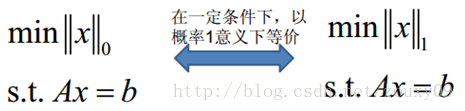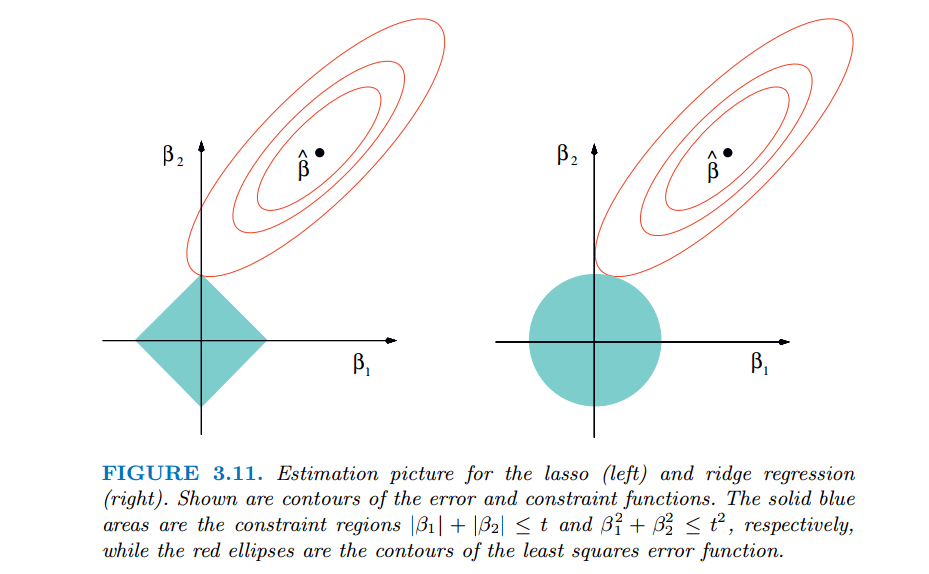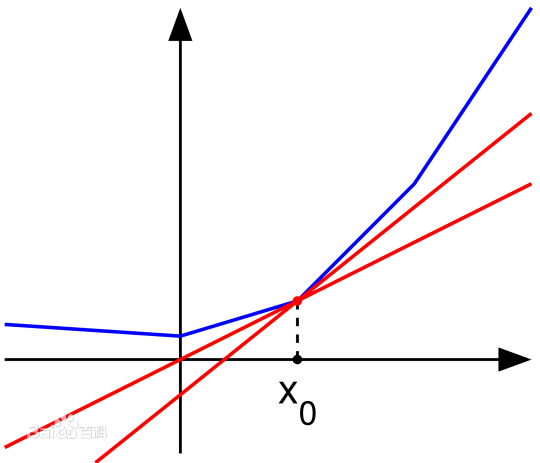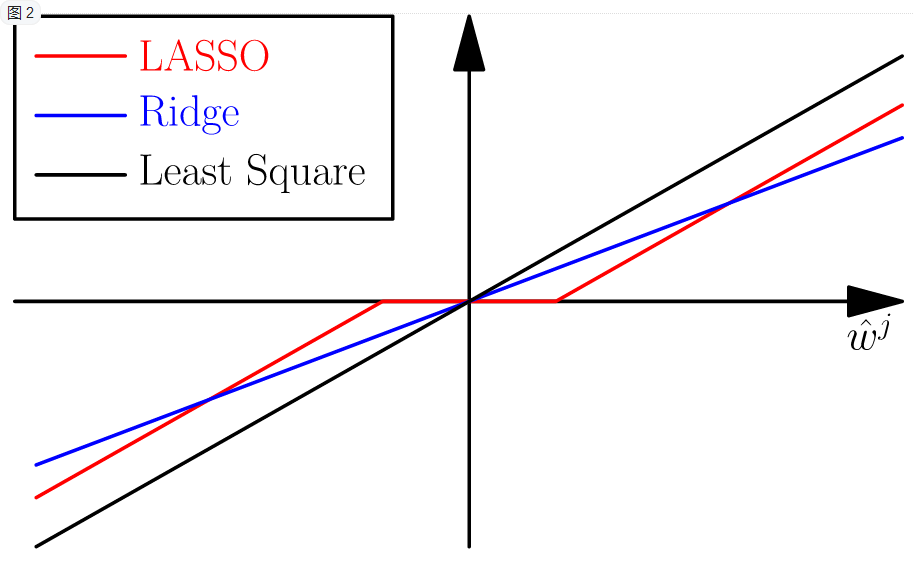# 机器学习方法：回归（二）：稀疏与正则约束ridge regression，Lasso

“机器学习方法“系列，我本着开放与共享（open and share）的精神撰写，目的是让更多的人了解机器学习的概念，理解其原理，学会应用。希望与志同道合的朋友一起交流，我刚刚设立了了一个技术交流QQ群：433250724，欢迎对算法、技术、应用感兴趣的同学加入，在交流中拉通——算法与技术，让理论研究与实际应用深度融合；也希望能有大牛能来，为大家解惑授业，福泽大众。推广开放与共享的精神。如果人多我就组织一些读书会，线下交流。

f(xi)=m=1pwmxim+w0=wTxi

J(w)=1ni=1n(yif(xi))2=1nyXw2

w=(XTX)1XTy

## ridge regression

JR(w)=12yXw2+λ2w2

w^R=(XTX+λI)1XTy

minw12yXw2,s.t.w2<θ

## 稀疏约束，Lasso

w2=(iwi2)1/2

w1=i|wi|

w0=i1(wi0)minw12yXw2,s.t.w1<θ## Lasso稀疏性的进一步理解：

JL(w)=12yXw2+λi|wi|w=XTy

JL(w)wjw¯j=0

(XTyXTXw¯)j+λsgn(w¯j)=0

w¯j=wjλsgn(w¯j)

w¯j=wjλsgn(w¯j)=sgn(wj)(|wj|λ)(|wj|λ)=|w¯j|0

w¯j=sgn(wj)(|wj|λ)+

0JL(w¯)=(XTyXTXw¯)+λe=w¯w+λe

|wj|=λ|ej|λ

w¯j=sgn(wj)(|wj|λ)+

OK，再回顾一下前面的ridge regression，如果也考虑上面说的orthonormal情况下，可以很容易得出最优解为

w^R=11+λw## 参考资料